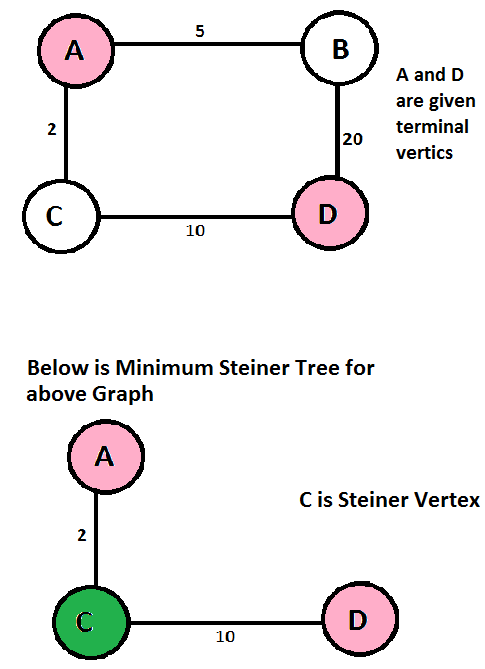# Steiner Tree Problem

• Difficulty Level : Hard
• Last Updated : 02 Jun, 2022

What is Steiner Tree? Given a graph and a subset of vertices in the graph, a Steiner tree spans through the given subset. The Steiner Tree may contain some vertices which are not in the given subset but are used to connect the vertices of the subset. The given set of vertices is called Terminal Vertices and other vertices that are used to construct the Steiner tree are called Steiner vertices. The Steiner Tree Problem is to find the minimum cost of Steiner Tree. See below for an example.Spanning Tree vs Steiner Tree Minimum Spanning Tree is a minimum weight tree that spans through all vertices. If the given subset (or terminal) vertices are equal to the set of all vertices in the Steiner Tree problem, then the problem becomes the Minimum Spanning Tree problem. And if the given subset contains only two vertices, then it shortest path problem between two vertices. Finding out Minimum Spanning Tree is polynomial-time solvable, but the Minimum Steiner Tree problem is NP-Hard and the related decision problem is NP-Complete. Applications of Steiner Tree Any situation where the task is to minimize the cost of connection among some important locations like VLSI Design, Computer Networks, etc. Shortest Path-based Approximate Algorithm Since the Steiner Tree problem is NP-Hard, there are no polynomial-time solutions that always give optimal cost. Therefore, there are approximate algorithms to solve the same. Below is one simple approximate algorithm.

```1) Start with a subtree T consisting of
one given terminal vertex
2) While T does not span all terminals
a) Select a terminal x not in T that is closest
to a vertex in T.
b) Add to T the shortest path that connects x with T```

The above algorithm is (2-2/n) approximate, i.e., it guarantees that the solution produced by this algorithm is not more than this ratio of optimized solution for a given graph with n vertices. There are better algorithms also that provide a better ratio. Refer to the below reference for more details. References: www.cs.uu.nl/docs/vakken/an/teoud/an-steiner.ppt This article is contributed by Shivam Gupta. Please write comments if you find anything incorrect, or if you want to share more information about the topic discussed above

My Personal Notes arrow_drop_up